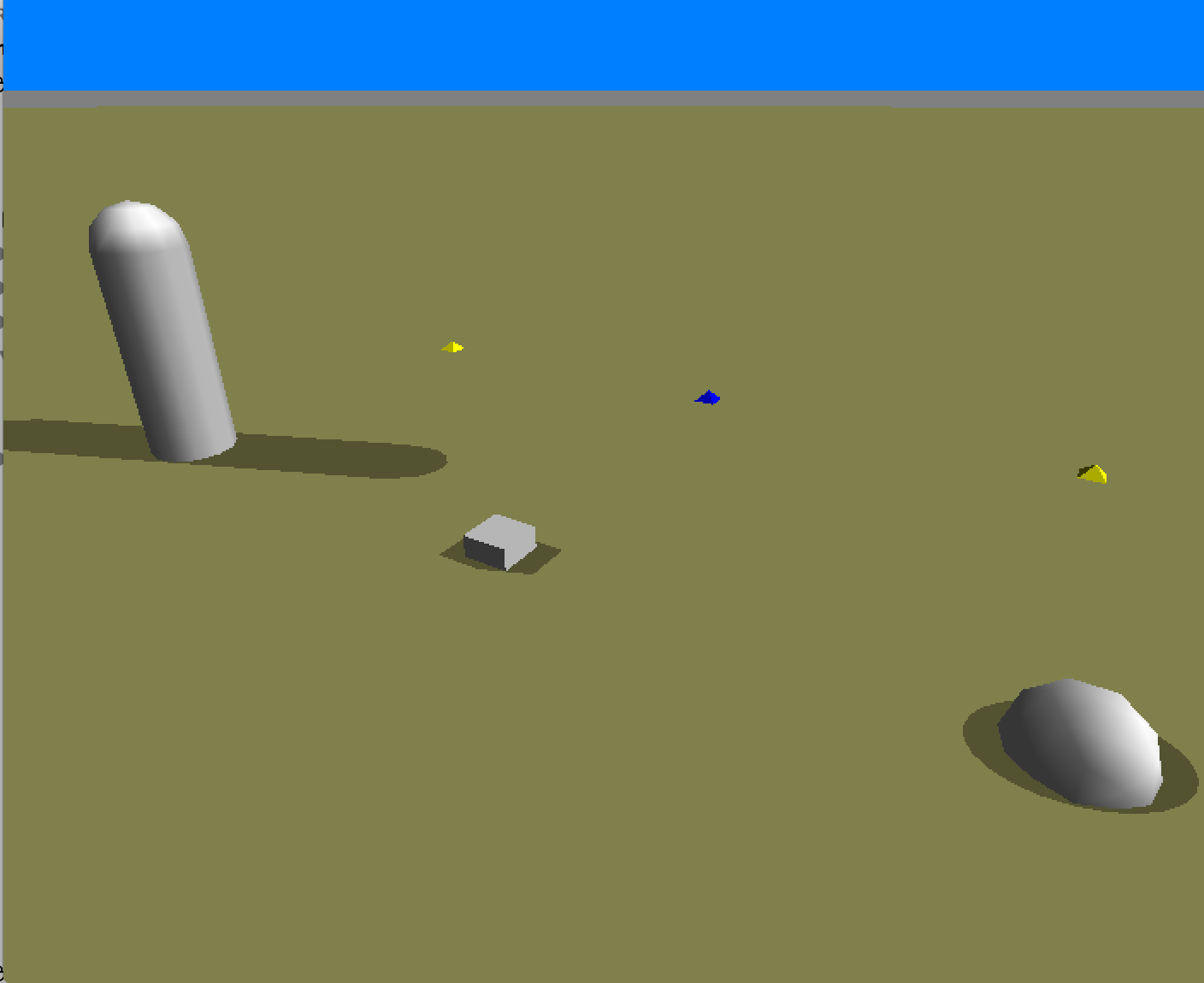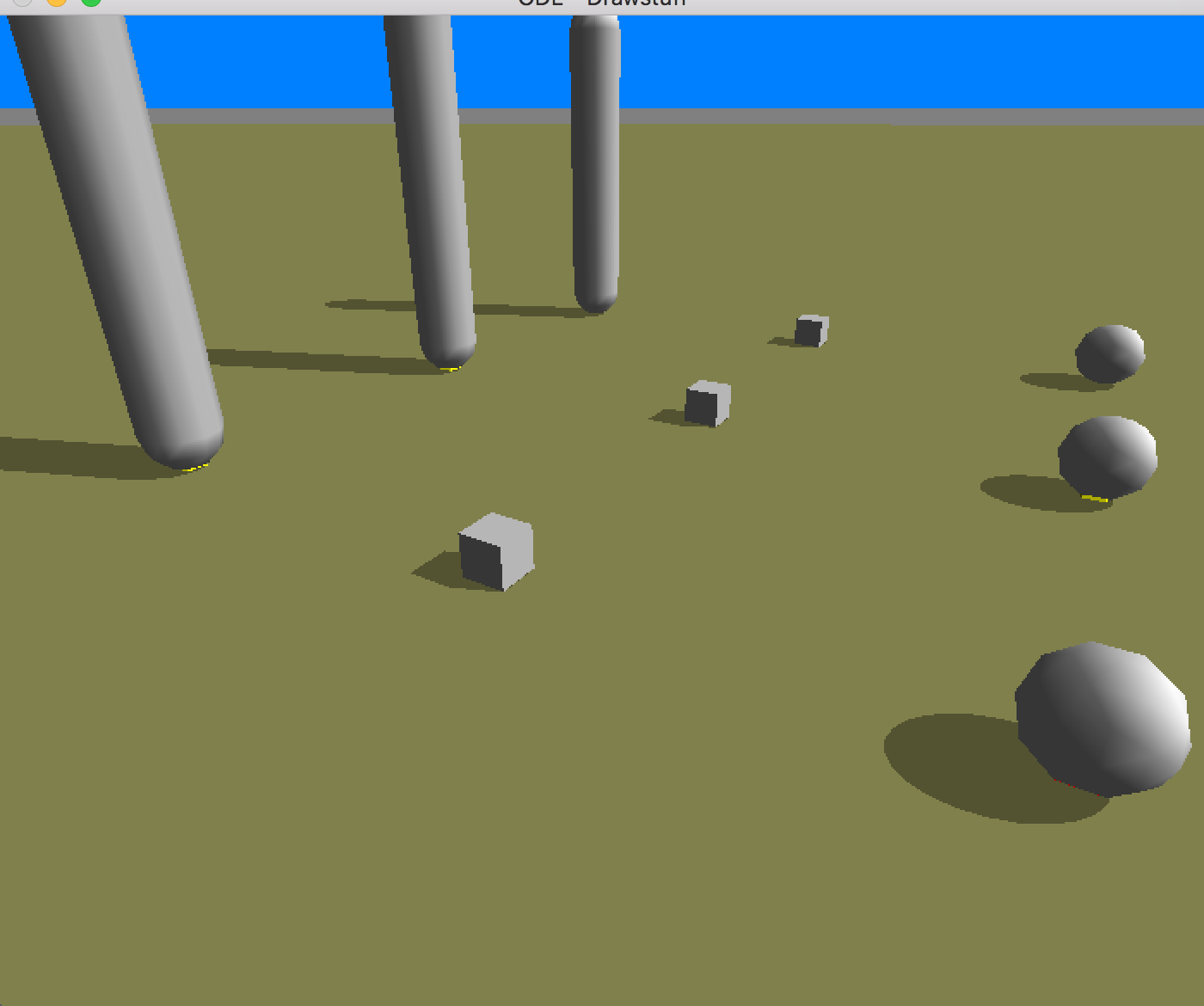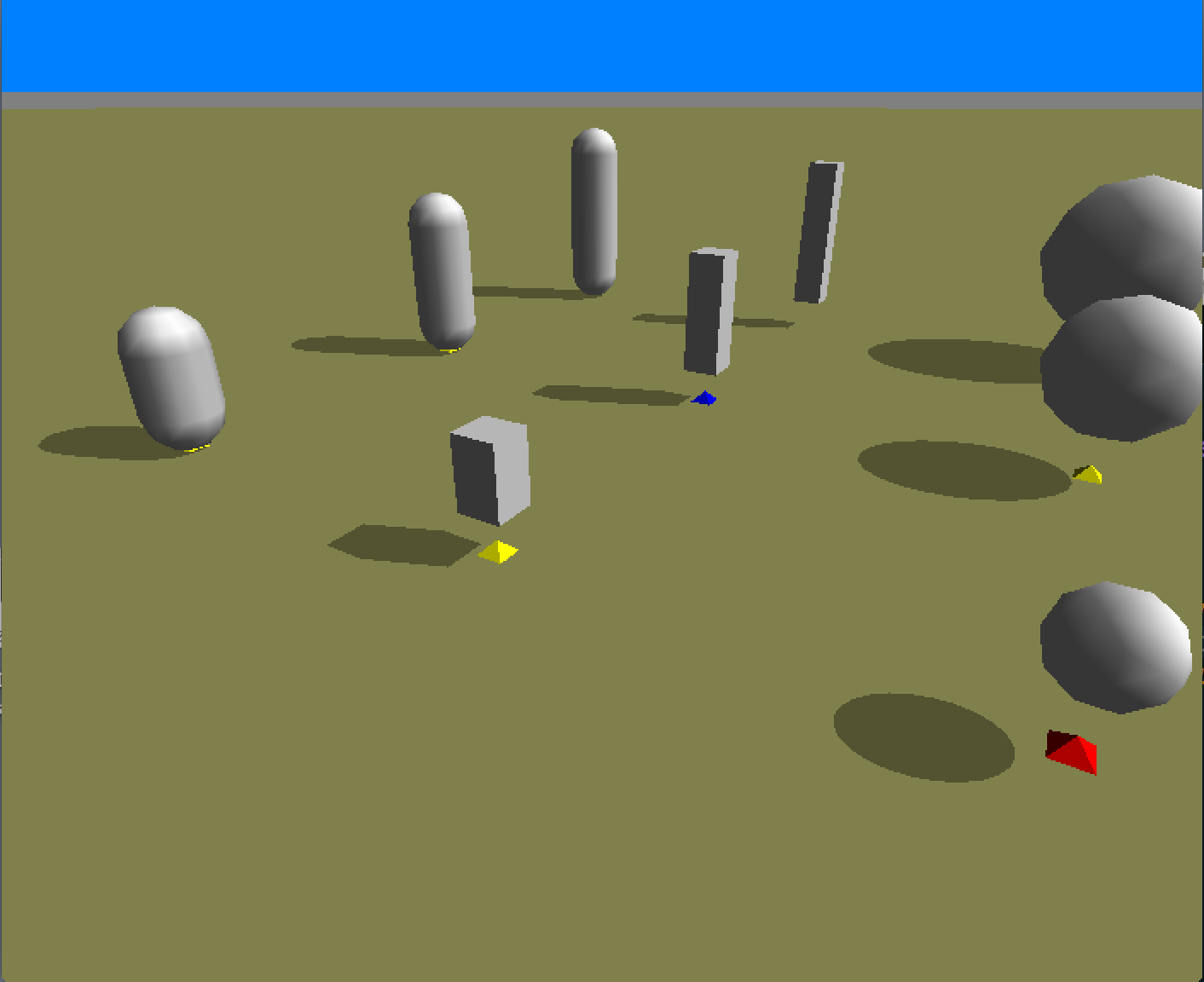# Bodies¶

## Basics¶

Pyrosim uses ODE to simulate rigid bodies. Rigid bodies cannot bend or deform and make hard contacts when they collide. Currently there are three types of rigid bodies in pyrosim:

1. Boxes
2. Cylinders
3. Spheres

This demo provides a quick introduction on the different bodies and how they are used in pyrosim.

## Sending bodies¶

The following code sends the default of each body shape at different x-coordinate positions.

sim = pyrosim.Simulator(play_paused=True)

sim.send_cylinder(x=-1)
sim.send_box(x=0)
sim.send_sphere(x=1)

sim.start()Note that when you play the simulation, because the bodies are embedded in the ground initially, they will shoot up. To rectify this we must change the objects z-coordinate.

## Body Position¶

We can also easily send multiple objects by using loops.

sim = pyrosim.Simulator(play_paused=True)

for i in range(3):
sim.send_cylinder(x=-1, y=i, z=.6,length=i)
sim.send_box(x=0, y=i, z=0.05)
sim.send_sphere(x=1, y=i, z=0.1)

sim.start()## Body Dimensions¶

Object dimensions can be set using the natural dimension parameters of each body.

sim = pyrosim.Simulator(play_paused=True)

for i in range(3):
size = (i+1)/6.0
z = size/2.0+0.1
sim.send_cylinder(x=-1, y=i, z=z,length=size)
sim.send_box(x=0, y=i, z=z, height=size)